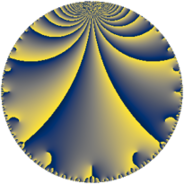# Properties

 Label 882.5.b.cLevel $882$ Weight $5$ Character orbit 882.b Analytic conductor $91.172$ Analytic rank $0$ Dimension $4$ CM no Inner twists $4$

# Related objects

## Newspace parameters

 Level: $$N$$ $$=$$ $$882 = 2 \cdot 3^{2} \cdot 7^{2}$$ Weight: $$k$$ $$=$$ $$5$$ Character orbit: $$[\chi]$$ $$=$$ 882.b (of order $$2$$, degree $$1$$, minimal)

## Newform invariants

 Self dual: no Analytic conductor: $$91.1723074400$$ Analytic rank: $$0$$ Dimension: $$4$$ Coefficient field: $$\Q(\sqrt{-2}, \sqrt{-53})$$ Defining polynomial: $$x^{4} - 52 x^{2} + 729$$ Coefficient ring: $$\Z[a_1, \ldots, a_{11}]$$ Coefficient ring index: $$2^{5}$$ Twist minimal: yes Sato-Tate group: $\mathrm{SU}(2)[C_{2}]$

## $q$-expansion

Coefficients of the $$q$$-expansion are expressed in terms of a basis $$1,\beta_1,\beta_2,\beta_3$$ for the coefficient ring described below. We also show the integral $$q$$-expansion of the trace form.

 $$f(q)$$ $$=$$ $$q -2 \beta_{1} q^{2} -8 q^{4} + \beta_{3} q^{5} + 16 \beta_{1} q^{8} +O(q^{10})$$ $$q -2 \beta_{1} q^{2} -8 q^{4} + \beta_{3} q^{5} + 16 \beta_{1} q^{8} + 2 \beta_{2} q^{10} + \beta_{1} q^{11} -2 \beta_{2} q^{13} + 64 q^{16} + 9 \beta_{3} q^{17} -11 \beta_{2} q^{19} -8 \beta_{3} q^{20} + 4 q^{22} -45 \beta_{1} q^{23} -223 q^{25} + 8 \beta_{3} q^{26} + 355 \beta_{1} q^{29} -31 \beta_{2} q^{31} -128 \beta_{1} q^{32} + 18 \beta_{2} q^{34} + 1560 q^{37} + 44 \beta_{3} q^{38} -16 \beta_{2} q^{40} -45 \beta_{3} q^{41} + 328 q^{43} -8 \beta_{1} q^{44} -180 q^{46} + 142 \beta_{3} q^{47} + 446 \beta_{1} q^{50} + 16 \beta_{2} q^{52} -2837 \beta_{1} q^{53} -\beta_{2} q^{55} + 1420 q^{58} -26 \beta_{3} q^{59} + 29 \beta_{2} q^{61} + 124 \beta_{3} q^{62} -512 q^{64} -1696 \beta_{1} q^{65} -1130 q^{67} -72 \beta_{3} q^{68} -299 \beta_{1} q^{71} -73 \beta_{2} q^{73} -3120 \beta_{1} q^{74} + 88 \beta_{2} q^{76} + 8650 q^{79} + 64 \beta_{3} q^{80} -90 \beta_{2} q^{82} -102 \beta_{3} q^{83} -7632 q^{85} -656 \beta_{1} q^{86} -32 q^{88} -457 \beta_{3} q^{89} + 360 \beta_{1} q^{92} + 284 \beta_{2} q^{94} -9328 \beta_{1} q^{95} -319 \beta_{2} q^{97} +O(q^{100})$$ $$\operatorname{Tr}(f)(q)$$ $$=$$ $$4q - 32q^{4} + O(q^{10})$$ $$4q - 32q^{4} + 256q^{16} + 16q^{22} - 892q^{25} + 6240q^{37} + 1312q^{43} - 720q^{46} + 5680q^{58} - 2048q^{64} - 4520q^{67} + 34600q^{79} - 30528q^{85} - 128q^{88} + O(q^{100})$$

Basis of coefficient ring in terms of a root $$\nu$$ of $$x^{4} - 52 x^{2} + 729$$:

 $$\beta_{0}$$ $$=$$ $$1$$ $$\beta_{1}$$ $$=$$ $$($$$$\nu^{3} - 25 \nu$$$$)/27$$ $$\beta_{2}$$ $$=$$ $$($$$$-4 \nu^{3} + 316 \nu$$$$)/27$$ $$\beta_{3}$$ $$=$$ $$4 \nu^{2} - 104$$
 $$1$$ $$=$$ $$\beta_0$$ $$\nu$$ $$=$$ $$($$$$\beta_{2} + 4 \beta_{1}$$$$)/8$$ $$\nu^{2}$$ $$=$$ $$($$$$\beta_{3} + 104$$$$)/4$$ $$\nu^{3}$$ $$=$$ $$($$$$25 \beta_{2} + 316 \beta_{1}$$$$)/8$$

## Character values

We give the values of $$\chi$$ on generators for $$\left(\mathbb{Z}/882\mathbb{Z}\right)^\times$$.

 $$n$$ $$199$$ $$785$$ $$\chi(n)$$ $$1$$ $$-1$$

## Embeddings

For each embedding $$\iota_m$$ of the coefficient field, the values $$\iota_m(a_n)$$ are shown below.

For more information on an embedded modular form you can click on its label.

Label $$\iota_m(\nu)$$ $$a_{2}$$ $$a_{3}$$ $$a_{4}$$ $$a_{5}$$ $$a_{6}$$ $$a_{7}$$ $$a_{8}$$ $$a_{9}$$ $$a_{10}$$
197.1
 −5.14782 + 0.707107i 5.14782 + 0.707107i 5.14782 − 0.707107i −5.14782 − 0.707107i
2.82843i 0 −8.00000 29.1204i 0 0 22.6274i 0 −82.3650
197.2 2.82843i 0 −8.00000 29.1204i 0 0 22.6274i 0 82.3650
197.3 2.82843i 0 −8.00000 29.1204i 0 0 22.6274i 0 82.3650
197.4 2.82843i 0 −8.00000 29.1204i 0 0 22.6274i 0 −82.3650
 $$n$$: e.g. 2-40 or 990-1000 Significant digits: Format: Complex embeddings Normalized embeddings Satake parameters Satake angles

## Inner twists

Char Parity Ord Mult Type
1.a even 1 1 trivial
3.b odd 2 1 inner
7.b odd 2 1 inner
21.c even 2 1 inner

## Twists

By twisting character orbit
Char Parity Ord Mult Type Twist Min Dim
1.a even 1 1 trivial 882.5.b.c 4
3.b odd 2 1 inner 882.5.b.c 4
7.b odd 2 1 inner 882.5.b.c 4
21.c even 2 1 inner 882.5.b.c 4

By twisted newform orbit
Twist Min Dim Char Parity Ord Mult Type
882.5.b.c 4 1.a even 1 1 trivial
882.5.b.c 4 3.b odd 2 1 inner
882.5.b.c 4 7.b odd 2 1 inner
882.5.b.c 4 21.c even 2 1 inner

## Hecke kernels

This newform subspace can be constructed as the intersection of the kernels of the following linear operators acting on $$S_{5}^{\mathrm{new}}(882, [\chi])$$:

 $$T_{5}^{2} + 848$$ $$T_{13}^{2} - 6784$$

## Hecke characteristic polynomials

$p$ $F_p(T)$
$2$ $$( 8 + T^{2} )^{2}$$
$3$ $$T^{4}$$
$5$ $$( 848 + T^{2} )^{2}$$
$7$ $$T^{4}$$
$11$ $$( 2 + T^{2} )^{2}$$
$13$ $$( -6784 + T^{2} )^{2}$$
$17$ $$( 68688 + T^{2} )^{2}$$
$19$ $$( -205216 + T^{2} )^{2}$$
$23$ $$( 4050 + T^{2} )^{2}$$
$29$ $$( 252050 + T^{2} )^{2}$$
$31$ $$( -1629856 + T^{2} )^{2}$$
$37$ $$( -1560 + T )^{4}$$
$41$ $$( 1717200 + T^{2} )^{2}$$
$43$ $$( -328 + T )^{4}$$
$47$ $$( 17099072 + T^{2} )^{2}$$
$53$ $$( 16097138 + T^{2} )^{2}$$
$59$ $$( 573248 + T^{2} )^{2}$$
$61$ $$( -1426336 + T^{2} )^{2}$$
$67$ $$( 1130 + T )^{4}$$
$71$ $$( 178802 + T^{2} )^{2}$$
$73$ $$( -9037984 + T^{2} )^{2}$$
$79$ $$( -8650 + T )^{4}$$
$83$ $$( 8822592 + T^{2} )^{2}$$
$89$ $$( 177103952 + T^{2} )^{2}$$
$97$ $$( -172586656 + T^{2} )^{2}$$